Courses

# Test: Electrochemistry- 2

## 30 Questions MCQ Test Chemistry for JEE | Test: Electrochemistry- 2

Description
This mock test of Test: Electrochemistry- 2 for Class 12 helps you for every Class 12 entrance exam. This contains 30 Multiple Choice Questions for Class 12 Test: Electrochemistry- 2 (mcq) to study with solutions a complete question bank. The solved questions answers in this Test: Electrochemistry- 2 quiz give you a good mix of easy questions and tough questions. Class 12 students definitely take this Test: Electrochemistry- 2 exercise for a better result in the exam. You can find other Test: Electrochemistry- 2 extra questions, long questions & short questions for Class 12 on EduRev as well by searching above.
QUESTION: 1

### The passage of current through a solution of certain electrolye results in the evolution of H2 at cathode and Cl2 at anode. The electrolytic solution is -

Solution:

In this Cl  will oxidise to give Cl2 ,Na+ reduction potential has lower potential than water reduction polenlid so water will reduce to give H2.

QUESTION: 2

### In an electrolytic cell current flows from -

Solution:

In an electrolytic cell, current flows from cathode to anode in outer circuit and in daniell cell, it is the reverse direction of flow of current from anode to cathode in outer circuit.

QUESTION: 3

### When an aqueous solution of H2SO4 is electrolysed, the ion discharged at anode is-

Solution:

Water oxidation at anode can be represented and SO42– can't be discharged
so
2H2O → 4H+ + O2 + 4e
so ion which will be discharged at anode will be OH.

QUESTION: 4

1 mole of Al is deposited by X coulomb of electricity passing through aluminium nitrate solution. The number of moles of silver deposited by X coulomb of electricity from silver nitrate solution is-

Solution:

Since to deposit 1 mole of aluminium 3 columb of electricity is required, as the alency of silver is + 1 so 3 mole of silver will be deposited by 3C of electricity

QUESTION: 5

A solution of Na2SO4 in water is electrolysed using Pt electrodes.The products at the cathode and anode are respectively -

Solution:

At cathode
4H2O + 4e → 4H2 + 4OH
At anode
2H2O → 4H+ + O2 + 4e
Thus at cathode we will get H2 whereas at anode we will get O2.

QUESTION: 6

In electrolysis of a fused salt, the weight deposited on an electrode will not depend on-

Solution:

In electrolysis of a fused salt, the weight deposited on electrode will not depend or temperature.

QUESTION: 7

The electrolysis of a solution resulted in the formation of H2 at the cathode and Cl2 at the anode. The liquid is-

Solution:

In this Cl will oxidise to give Cl2 Na+ water reduction potential has higher potential than that of water reduction potential, so water will reduce to give H2.

QUESTION: 8

Which loses charge at cathode-

Solution:

Cation loses charge at cathode as it gave electron

QUESTION: 9

In the electrolysis of CuSO4, the reaction :

Cu2+ + 2e¯ → Cu, takes place at :

Solution:

As reduction will take place at cathode so reaction Cu2+ + 2e → Cu
Will take place at cathode

QUESTION: 10

If mercury is used as cathode in the electrolysis of aqueous NaCl solution, the ions discharged at cathode are-

Solution:

If mercury  is used as cathode in the electrolysis of aqueos NaCl solution then the metal is discharged at mercury to from amalgam.

QUESTION: 11

The specific conductance of a solution is 0.3568 ohm-1. When placed in a cell the conductance is 0.0268 ohm-1. The cell constant is-

Solution:

K = 0.3568 W cm–1
condutance = 0.0268  Ω –1
K = G ×1/A
0.3568 = 0.0268 ×1/9
13.31 cm–1

QUESTION: 12

A conductance cell was filled with a 0.02 M KCl solution which has a specific conductance of 2.768 × 10-3 ohm-1 cm-1. If its resistance is 82.4 ohm at 25ºC, the cell constant is-

Solution:

K = G. L/A
10–3 × 2.768 = 1/R ×L/A
L/A = 228.08 × 10–3
= 0.2281 cm–1

QUESTION: 13

The variation of equivalent conductance vs decrease in concentration of a strong electrolyte is correctly given in the plot -

Solution: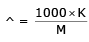On decreasing the value of M will increase but increase will be hyberbolic.

QUESTION: 14

Which of the following solutions has the highest equivalent conductance ?

Solution:

Higher the dilution higher will be the equivalent conductance

QUESTION: 15

The resistance of 0.01N solution of an electrolyte AB at 328K is 100 ohm. The specific conductance of solution is (cell constant = 1cm-1) -

Solution: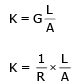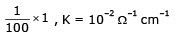QUESTION: 16

For an electrolytic solution of 0.05 mol L-1, the conductivity has been found to be 0.0110 Scm-1.The molar conductivity is-

Solution: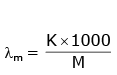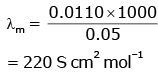QUESTION: 17

Two electrodes are fitted in conductance cell 1.5 cm apart while the area of cross section of each electrode is 0.75 cm2. The cell constant is-

Solution:

L/A is cell constant.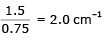QUESTION: 18

The best conductor of electricity is in 1M solution of-

Solution:

That electrolyte will be the best electrolyte which has maximum number of ions.

QUESTION: 19

A certain current liberates 0.504 g of H2 in 2 hours. How many grams of copper can be liberated by the same current flowing for the same time in CuSO4 solution -

Solution:

gm eq.  of H2 = gm eq. of Cu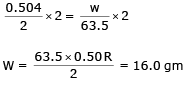QUESTION: 20

A currnet of 2.6 ampere is passed through CuSO4 solution for 6 minutes 20 seconds. The amount of Cu deposited is (At. wt. of Cu = 63.5, Faraday = 96500 C)-

Solution: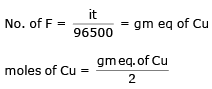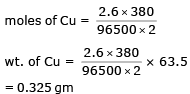QUESTION: 21

Three Faradays of electricity are passed through molten Al2O3, aqueous solution of CuSO4 and molten NaCl taken in three different electrolytic cells. The amount of Al, Cu and Na deposited at the cathodes will be in the ratio of-

Solution:

gm equivalent of Al = gm eq. of Cu = gm eq. of Na
3 = 3 = 3
3/3/ = 3/2 = 1
1 : 1.5 : 3

QUESTION: 22

The quantity of electricity required to liberate 0.01g equivalent of an element at the electrode is-

Solution: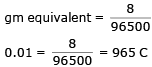QUESTION: 23

The unit of electrochemical equivalent is-

Solution:

Z = w/it
w in gm
it in columb.
so z = gm/columb

QUESTION: 24

One faraday of electricity will liberate one mole of metal from a solution of-

Solution:

Since KCl has the n-factor of 1 so 1 faraday of electricity will liberate one mole of metal from a solution.

QUESTION: 25

The number of faraday required to generate 1 mole of Mg from MgCl2 is-

Solution:

Since magnesium has the n-factor 2 so the number of faraday rquired to genereate 1 mole of Mg will be 2 .

QUESTION: 26

One gm metal M+2 was discharged by the passage of 1.81×1022 electrons. What is the atomic weight of metal?

Solution: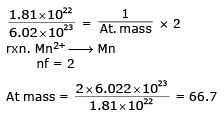QUESTION: 27

One mole of electron passes through each of the solution of AgNO3, CuSO4 and AlCl3 when Ag, Cu and Al are deposited at cathode. The molar ratio of Ag, Cu and Al deposited are

Solution:

Molar ratio
All have the same equivalent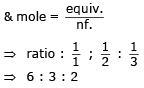QUESTION: 28

Salts of A (atomic weight = 7), B (atomic weight = 27) and C (atomic weight = 48) were electrolysed under identical conditions using the same quantity of electricity. It was found that when 2.1 g of A was deposited, the weights of B and C deposited were 2.7 and 7.2 g. The valencies of A, B and C respectively are

Solution: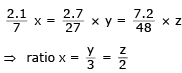If x = 1 ⇒ y = 3, z = 2

QUESTION: 29

The density of Cu is 8.94 g cm–3. The quantity of electricity needed to plate an area 10 cm × 10cm to a thickness of 10-2 cm using CuSO4 solution would be

Solution:

Volume : 10 × 10 × 10–2 = 1 cm3
mass of Cu = 8.94 g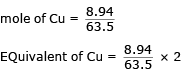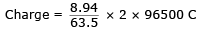= 27172 C

QUESTION: 30

During electrolysis of an aqueous solution of sodium sulphate if 2.4 L of oxygen at STP was liberated at anode. The volume of hydrogen at STP, liberated at cathode would be :

Solution:

Equivalent of H2 = Equivalent of O2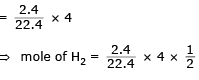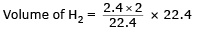= 4.8 L